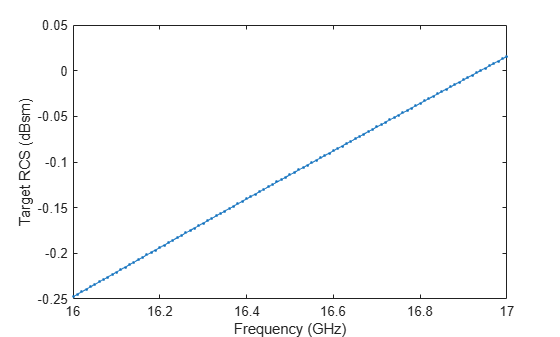# sarSurfaceRCS

Radar cross-section of target for SAR

Since R2021a

## Syntax

``rcs = sarSurfaceRCS(sigmaref,freq,freqref,rngazres,grazang)``
``rcs = sarSurfaceRCS(sigmaref,freq,freqref,rngazres,grazang,n)``
``rcs = sarSurfaceRCS(nrcs,rngazres,grazang)``

## Description

example

````rcs = sarSurfaceRCS(sigmaref,freq,freqref,rngazres,grazang)` returns the target radar cross-section (RCS) for SAR as projected on the ground.```

example

````rcs = sarSurfaceRCS(sigmaref,freq,freqref,rngazres,grazang,n)` specifies a frequency-dependent proportionality factor that depends upon the target characteristics.```
````rcs = sarSurfaceRCS(nrcs,rngazres,grazang)` uses as input the surface normalized radar cross-section, also known as the reflectivity or σ0.```

## Examples

collapse all

Estimate the target radar cross-section (RCS) of a side-looking airborne SAR operating at frequencies between 16 GHz to 17 GHz and grazing at 30${}^{\circ }$. The target reflectivity is –25 dB at the Ku band (nominally 16.7 GHz). The radar has a slant range resolution of 15 m and an azimuth resolution of 18 m. Assume a frequency-dependent proportionality factor of 1.

```f = 16e9:1e7:17e9; sigmaref = -25; fref = 16.7e9; rngazres = [15 18]; grazang = 30;```

Convert the reflectivity to linear units. Compute the target RCS.

`sigma = sarSurfaceRCS(db2pow(sigmaref),f,fref,rngazres,grazang);`

Plot the RCS in decibels as a function of frequency.

```plot(f/1e9,pow2db(sigma),'.-') xlabel('Frequency (GHz)') ylabel('Target RCS (dBsm)')```## Input Arguments

collapse all

Reflectivity at nominal reference frequency in square meters per square meter, specified as a positive real scalar.

Data Types: `double`

Radar frequency in hertz, specified as a positive real scalar or a vector.

Data Types: `double`

Nominal reference frequency in hertz, specified as a positive real scalar.

Data Types: `double`

Slant range and azimuth resolutions, specified as a 1-by-2 row vector of positive real scalars.

• The first element of `rngazres` specifies the slant range resolution in meters.

• The second element of `rngazres` specifies the azimuth or cross-range resolution in meters.

Data Types: `double`

Grazing angle in degrees, specified as a scalar in the range [`0`, `90`].

Data Types: `double`

Frequency-dependent proportionality factor, specified as a real scalar. For distributed targets, `n` varies between 0 and 1. For nondistributed targets, `n` is a positive real scalar.

Data Types: `double`

Surface normalized radar cross-section in square meters per square meter, specified as a nonnegative scalar or row vector. The surface normalized radar cross-section is also known as the reflectivity or σ0.

Data Types: `double`

## Output Arguments

collapse all

Target radar cross-section for SAR as projected on the ground in square meters, returned as a scalar or a vector. `rcs` has the same dimensions as either `freq` or `nrcs`.

## Version History

Introduced in R2021a